# 0/1 Knapsack (DP – 19)

Problem Statement: 0/1 Knapsack

Problem Link: 0/1 Knapsack

A thief wants to rob a store. He is carrying a bag of capacity W. The store has ‘n’ items. Its weight is given by the ‘wt’ array and its value by the ‘val’ array. He can either include an item in its knapsack or exclude it but can’t partially have it as a fraction. We need to find the maximum value of items that the thief can steal.

```Explanation: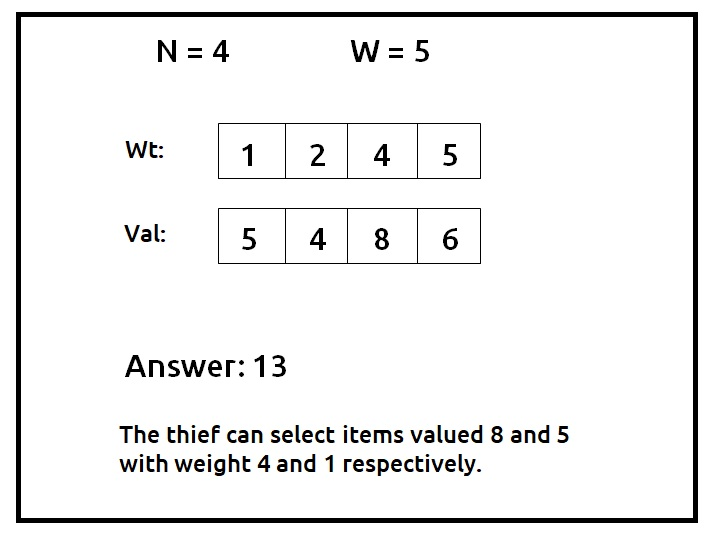```

Disclaimer: Don’t jump directly to the solution, try it out yourself first.

Pre-req: Count Subsets with Sum K

### Solution :

Why a Greedy Solution doesn’t work?

The first approach that comes to our mind is greedy. A greedy solution will fail in this problem because there is no ‘uniformity’ in data. While selecting a local better choice we may choose an item that will in long term give less value.

Let us understand this with help of an example: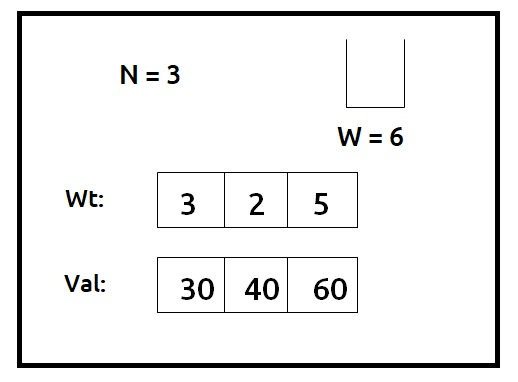A Greedy solution will be to take the most valuable item first, so we will take an item on index 2, with a value of 60, and put it in the knapsack. Now the remaining capacity of the knapsack will be 1. Therefore we cannot add any other item. So a greedy solution gives us the answer 60.

Now we can clearly see that a non-greedy solution of taking the first two items will give us the value of 70 (30+40) in the given capacity of the knapsack.

As the greedy approach doesn’t work, we will try to generate all possible combinations using recursion and select the combination which gives us the maximum value in the given constraints.

Steps to form the recursive solution:

We will first form the recursive solution by the three points mentioned in Dynamic Programming Introduction

Step 1: Express the problem in terms of indexes.

We are given ‘n’ items. Their weight is represented by the ‘wt’ array and value by the ‘val’ array. So clearly one parameter will be ‘ind’, i.e index up to which the array items are being considered.

There is one more parameter “W”. We need the capacity of the knapsack to decide whether we can pick an array item or not in the knapsack.

So, we can say that initially, we need to find f(n-1, W) where W is the overall capacity given to us. f(n-1, W) means we are finding the maximum value of items that the thief can steal from items with index 0 to n-1 capacity W of the knapsack.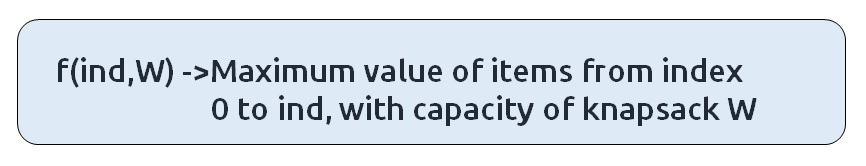Base Cases:

• If ind==0, it means we are at the first item, so in that case we will check whether this item’s weight is less than or equal to the current capacity W, if it is, we simply return its value (val) else we return 0.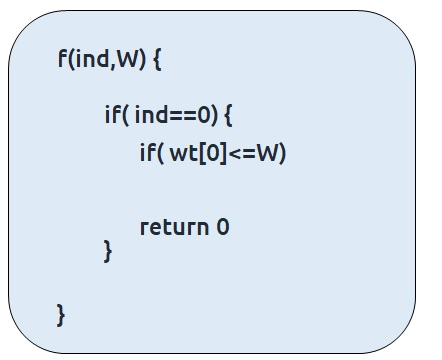Step 2: Try out all possible choices at a given index.

We need to generate all the subsequences. We will use the pick/non-pick technique as discussed in this video “Recursion on Subsequences”.

We have two choices:

• Exclude the current element in the subsequence: We first try to find a subsequence without considering the current index item. If we exclude the current item, the capacity of the bag will not be affected and the value added will be 0 for the current item. So we will call the recursive function f(ind-1,W)
• Include the current element in the subsequence: We will try to find a subsequence by considering the current item to the knapsack. As we have included the item, the capacity of the knapsack will be updated to W-wt[ind] and the current item’s value (val[ind] will also be added to the further recursive call answer. We will make a recursive call to f(ind-1, W- wt[ind]).

Note: We will consider the current item in the subsequence only when the current element’s weight is less than or equal to the capacity ‘W’ of the knapsack, if it isn’t we will not be considering it.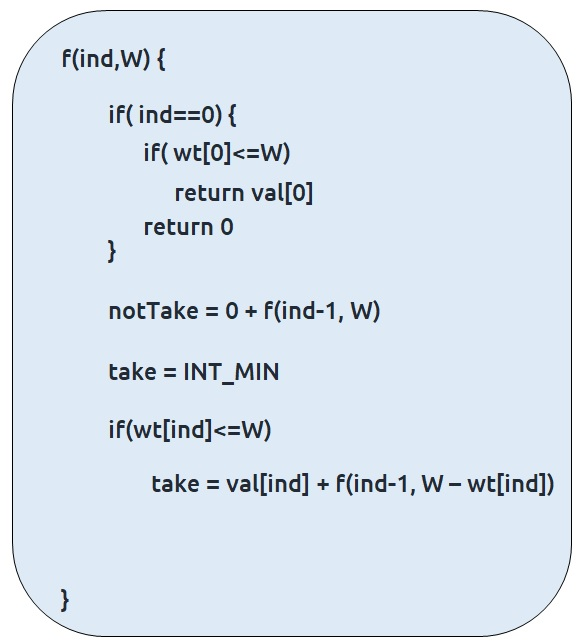Step 3:  Return the maximum of take and notTake

As we have to return the maximum amount of value, we will return the max of take and notTake as our answer.

The final pseudocode after steps 1, 2, and 3: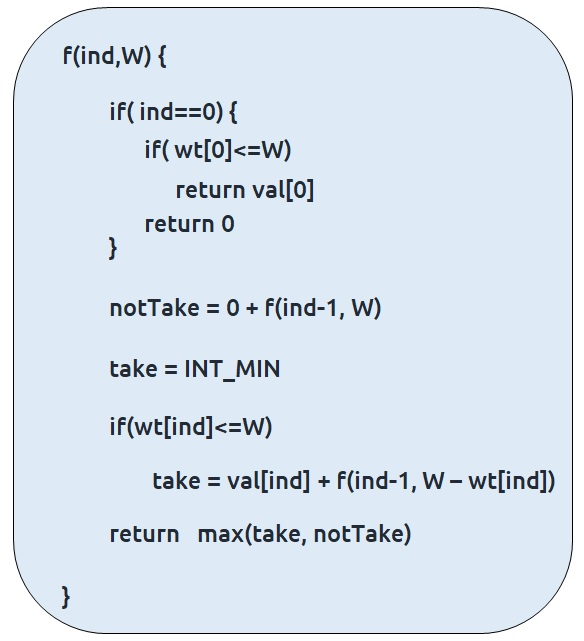Steps to memoize a recursive solution:

If we draw the recursion tree, we will see that there are overlapping subproblems. In order to convert a recursive solution the following steps will be taken:

1. Create a dp array of size [n][W+1]. The size of the input array is ‘N’, so the index will always lie between ‘0’ and ‘n-1’. The capacity can take any value between ‘0’ and ‘W’. Therefore we take the dp array as dp[n][W+1]
2. We initialize the dp array to -1.
3. Whenever we want to find the answer of particular parameters (say f(ind,target)), we first check whether the answer is already calculated using the dp array(i.e dp[ind][target]!= -1 ). If yes, simply return the value from the dp array.
4. If not, then we are finding the answer for the given value for the first time, we will use the recursive relation as usual but before returning from the function, we will set dp[ind][target] to the solution we get.

Code:

## C++ Code

``````#include <bits/stdc++.h>

using namespace std;

int knapsackUtil(vector<int>& wt, vector<int>& val, int ind, int W, vector<vector<int>>& dp){

if(ind == 0){
if(wt <=W) return val;
else return 0;
}

if(dp[ind][W]!=-1)
return dp[ind][W];

int notTaken = 0 + knapsackUtil(wt,val,ind-1,W,dp);

int taken = INT_MIN;
if(wt[ind] <= W)
taken = val[ind] + knapsackUtil(wt,val,ind-1,W-wt[ind],dp);

return dp[ind][W] = max(notTaken,taken);
}

int knapsack(vector<int>& wt, vector<int>& val, int n, int W){

vector<vector<int>> dp(n,vector<int>(W+1,-1));
return knapsackUtil(wt, val, n-1, W, dp);
}

int main() {

vector<int> wt = {1,2,4,5};
vector<int> val = {5,4,8,6};
int W=5;

int n = wt.size();

cout<<"The Maximum value of items, thief can steal is " <<knapsack(wt,val,n,W);
}
``````

Output:

The Maximum value of items, thief can steal is 13

Time Complexity: O(N*W)

Reason: There are N*W states therefore at max ‘N*W’ new problems will be solved.

Space Complexity: O(N*W) + O(N)

Reason: We are using a recursion stack space(O(N)) and a 2D array ( O(N*W)).

## Java Code

``````import java.util.*;

class TUF{
static int knapsackUtil(int[] wt,int[] val, int ind, int W,int[][] dp){

if(ind == 0){
if(wt <=W) return val;
else return 0;
}

if(dp[ind][W]!=-1)
return dp[ind][W];

int notTaken = 0 + knapsackUtil(wt,val,ind-1,W,dp);

int taken = Integer.MIN_VALUE;
if(wt[ind] <= W)
taken = val[ind] + knapsackUtil(wt,val,ind-1,W-wt[ind],dp);

return dp[ind][W] = Math.max(notTaken,taken);
}

static int knapsack(int[] wt,int[] val, int n, int W){

int dp[][]= new int[n][W+1];
for(int row[]: dp)
Arrays.fill(row,-1);
return knapsackUtil(wt, val, n-1, W, dp);
}

public static void main(String args[]) {

int wt[] = {1,2,4,5};
int val[] = {5,4,8,6};
int W=5;

int n = wt.length;

System.out.println("The Maximum value of items, thief can steal is "+
knapsack(wt,val,n,W));
}
}``````

Output:

The Maximum value of items, thief can steal is 13

Time Complexity: O(N*W)

Reason: There are N*W states therefore at max ‘N*W’ new problems will be solved.

Space Complexity: O(N*W) + O(N)

Reason: We are using a recursion stack space(O(N)) and a 2D array ( O(N*W)).

Steps to convert Recursive Solution to Tabulation one.

To convert the memoization approach to a tabulation one, create a dp array with the same size as done in memoization. We can initialize it as 0.

First, we need to initialize the base conditions of the recursive solution.

• At ind==0, we are considering the first element, if the capacity of the knapsack is greater than the weight of the first item, we return val as answer. We will achieve this using a for loop.
• Next, we are done for the first row, so our ‘ind’ variable will move from 1 to n-1, whereas our ‘cap’ variable will move from 0 to ‘W’. We will set the nested loops to traverse the dp array.
• Inside the nested loops we will apply the recursive logic to find the answer of the cell.
• When the nested loop execution has ended, we will return dp[n-1][W] as our answer.

Code:

## C++ Code

``````#include <bits/stdc++.h>

using namespace std;

int knapsack(vector<int>& wt, vector<int>& val, int n, int W){

vector<vector<int>> dp(n,vector<int>(W+1,0));

//Base Condition

for(int i=wt; i<=W; i++){
dp[i] = val;
}

for(int ind =1; ind<n; ind++){
for(int cap=0; cap<=W; cap++){

int notTaken = 0 + dp[ind-1][cap];

int taken = INT_MIN;
if(wt[ind] <= cap)
taken = val[ind] + dp[ind-1][cap - wt[ind]];

dp[ind][cap] = max(notTaken, taken);
}
}

return dp[n-1][W];
}

int main() {

vector<int> wt = {1,2,4,5};
vector<int> val = {5,4,8,6};
int W=5;

int n = wt.size();

cout<<"The Maximum value of items, thief can steal is " <<knapsack(wt,val,n,W);
}
``````

Output:

The Maximum value of items, thief can steal is 13

Time Complexity: O(N*W)

Reason: There are two nested loops

Space Complexity: O(N*W)

Reason: We are using an external array of size ‘N*W’. Stack Space is eliminated.

## Java Code

``````import java.util.*;

class TUF{
static int knapsack(int[] wt,int[] val, int n, int W){

int dp[][] = new int[n][W+1];

//Base Condition

for(int i=wt; i<=W; i++){
dp[i] = val;
}

for(int ind =1; ind<n; ind++){
for(int cap=0; cap<=W; cap++){

int notTaken = 0 + dp[ind-1][cap];

int taken = Integer.MIN_VALUE;
if(wt[ind] <= cap)
taken = val[ind] + dp[ind-1][cap - wt[ind]];

dp[ind][cap] = Math.max(notTaken, taken);
}
}

return dp[n-1][W];
}

public static void main(String args[]) {

int wt[] = {1,2,4,5};
int val[] = {5,4,8,6};
int W=5;

int n = wt.length;

System.out.println("The Maximum value of items, thief can steal is "
+knapsack(wt,val,n,W));
}
}``````

Output:

The Maximum value of items, thief can steal is 13

Time Complexity: O(N*W)

Reason: There are two nested loops

Space Complexity: O(N*W)

Reason: We are using an external array of size ‘N*W’. Stack Space is eliminated.

Part 3: Space Optimization

If we closely look the relation,

dp[ind][cap] =  max(dp[ind-1][cap] ,dp[ind-1][cap-wt[ind]]

We see that to calculate a value of a cell of the dp array, we need only the previous row values (say prev). So, we don’t need to store an entire array. Hence we can space optimize it.

We will be space optimizing this solution using only one row.

Intuition:

If we closely observe, we fill in the following manner in two-row space optimization:

• We will  initialize the first row and then using its values we will the next row.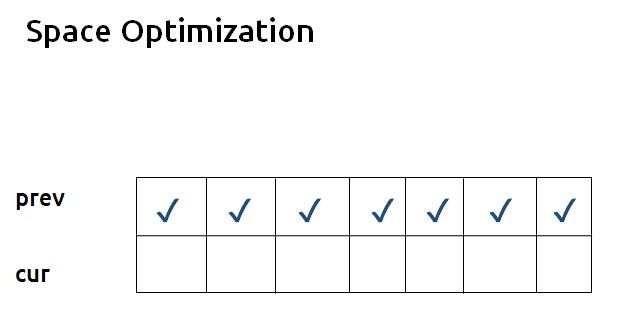• If we clearly see the values required:  dp[ind-1][cap] and dp[ind-1][cap – wt[ind]], we can say that if we are at a column cap, we will only require the values shown in the green region and none in the red region shown in the below image ( because cap – wt[ind] will always be less than the cap).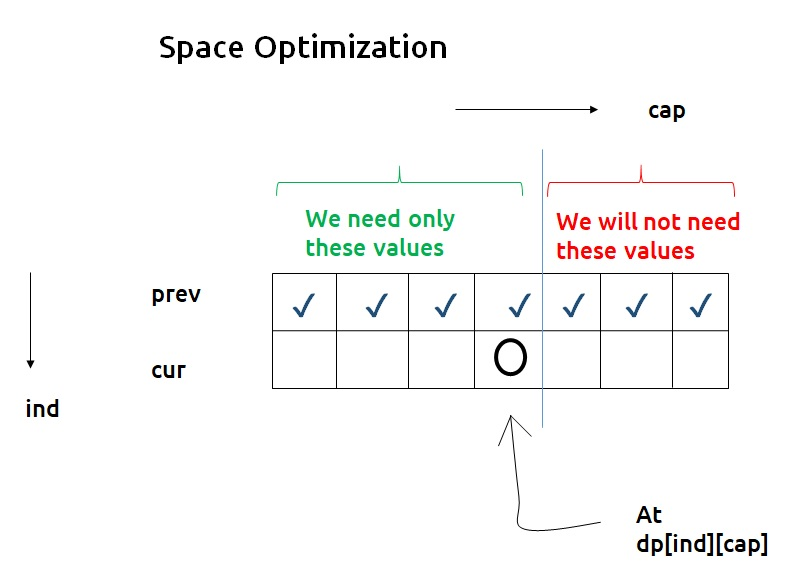• As we don’t want values from the right, we can start filling this new row from the right rather than the left.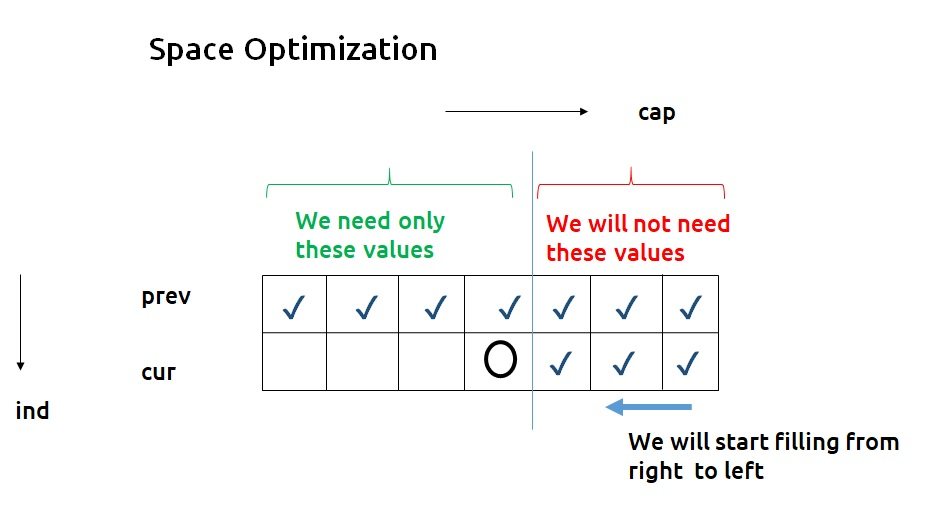• Now here is the catch, if we are filling from the right and at any time we need the previous row’s value of the leftward columns only, why do we need to have two rows in the first place? We can use a single row and overwrite the new computed values on itself in order to store it.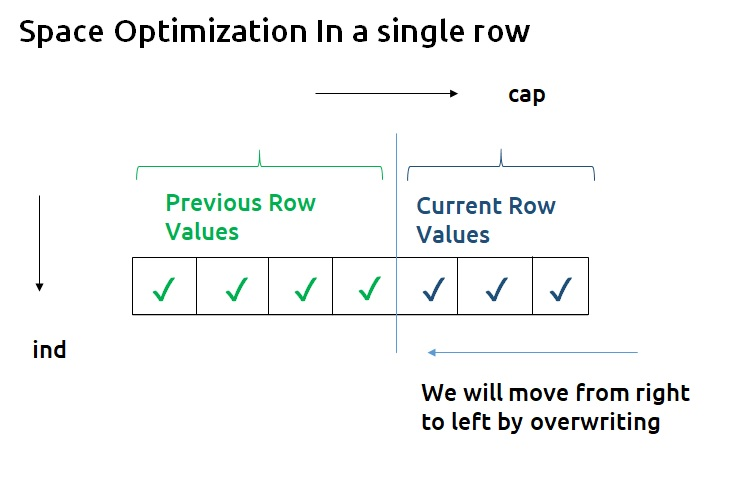Code:

## C++ Code

``````#include <bits/stdc++.h>

using namespace std;

int knapsack(vector<int>& wt, vector<int>& val, int n, int W){

vector<int> prev(W+1,0);

//Base Condition

for(int i=wt; i<=W; i++){
prev[i] = val;
}

for(int ind =1; ind<n; ind++){
for(int cap=W; cap>=0; cap--){

int notTaken = 0 + prev[cap];

int taken = INT_MIN;
if(wt[ind] <= cap)
taken = val[ind] + prev[cap - wt[ind]];

prev[cap] = max(notTaken, taken);
}
}

return prev[W];
}

int main() {

vector<int> wt = {1,2,4,5};
vector<int> val = {5,4,8,6};
int W=5;

int n = wt.size();

cout<<"The Maximum value of items, thief can steal is " <<knapsack(wt,val,n,W);
}
``````

Output:

The Maximum value of items, thief can steal is 13

Time Complexity: O(N*W)

Reason: There are two nested loops.

Space Complexity: O(W)

Reason: We are using an external array of size ‘W+1’ to store only one row.

## Java Code

``````import java.util.*;

class TUF{
static int knapsack(int[] wt,int[] val, int n, int W){

int prev[]=new int[W+1];

//Base Condition

for(int i=wt; i<=W; i++){
prev[i] = val;
}

for(int ind =1; ind<n; ind++){
for(int cap=W; cap>=0; cap--){

int notTaken = 0 + prev[cap];

int taken = Integer.MIN_VALUE;
if(wt[ind] <= cap)
taken = val[ind] + prev[cap - wt[ind]];

prev[cap] = Math.max(notTaken, taken);
}
}

return prev[W];
}

public static void main(String args[]) {

int wt[] = {1,2,4,5};
int val[] = {5,4,8,6};
int W=5;

int n = wt.length;

System.out.println("The Maximum value of items, thief can steal is " +knapsack(wt,val,n,W));
}
}``````

Output:

The Maximum value of items, thief can steal is 13

Time Complexity: O(N*W)

Reason: There are two nested loops.

Space Complexity: O(W)

Reason: We are using an external array of size ‘W+1’ to store only one row.

Special thanks to Anshuman Sharma for contributing to this article on takeUforward. If you also wish to share your knowledge with the takeUforward fam, please check out this article